# Problem Statement :

You are given a three integers X,N, and M. Your task is to find (X^N) % M. A^B is defined as A raised to power B and A%M is the remainder when A is divided by M.

Sample Input :

`3 1 2`

Sample Output :

`1`

Explanation :

X = 3, N = 1, and M = 2
X^N = 3¹ = 3
X^N %M = 3 % 2 = 1.
So the answer will be 1.

Sample Input :

`4 3 10`

Sample Output :

`4`

Explanation :

X = 4, N = 3, and M = 10
X^N = 4³ = 64
X^N %M = 64 % 10 = 4.
So the answer will be 4.

# Iterative Approach

In this solution, we will use binary exponentiation.

The idea here is to split N in powers of two by converting it into its binary representation to achieve answer in O(logN) steps.

For example if N = 7 and X = 8

The binary representation of N = 111

So 8⁷ can be calculated using multiplication 8⁴ , 8², 8

So in each step, we will keep squaring X and if N has a set bit its binary representation then we will multiply X to answer.

Steps :

Time Complexity : O(log N)
Space Complexity : O(1)

# Code :

Placewit grows the best engineers by providing an interactive classroom experience and by helping them develop their skills and get placed in amazing companies.

--

--

--

## More from Placewit

Upskilling students for tech placements!

Love podcasts or audiobooks? Learn on the go with our new app.

## Raspberry PI 4 and Docker Series Part I - Setting up the core requirements## GitHub Crash Course #1 Introduction to GitHub## [Leetcode] Reverse Nodes in k-Group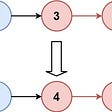## Beginner Guide: Running a ChainLink Node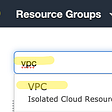## Drawing Die Faces with SwiftUI-Part 3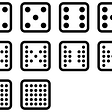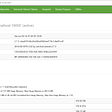## Getting started with Dockerizing your Node.js Application## Placewit

Upskilling students for tech placements!

## Google Interview Question — LeetCode 1423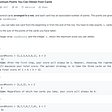## Minimum Moves to Reach Target Score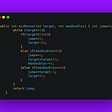## Color of Next Ball Puzzle## The Blind 75 Leetcode Series: Merge Two Sorted Lists